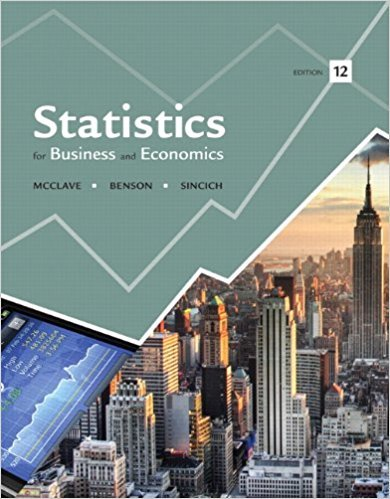×
Log in to StudySoup
Get Full Access to Statistics For Business And Economics - 12 Edition - Chapter 8 - Problem 5e
Join StudySoup for FREE
Get Full Access to Statistics For Business And Economics - 12 Edition - Chapter 8 - Problem 5e

Already have an account? Login here
×
Reset your password

# Two populations are described in each of the followingISBN: 9780321826237 51

## Solution for problem 5E Chapter 8

Statistics for Business and Economics | 12th Edition

• Textbook Solutions
• 2901 Step-by-step solutions solved by professors and subject experts
• Get 24/7 help from StudySoup virtual teaching assistantsStatistics for Business and Economics | 12th Edition

4 5 1 342 Reviews
18
3
Problem 5E

Problem 5E

Two populations are described in each of the following cases. In which cases would it be appropriate to apply the small-sample t-test to investigate the difference between the population means?

a. Population 1: Normal distribution with varianceb. Population 1: Normal distribution with variancec. Population 1: Skewed to the left with varianced. Population 1: Normal distribution with variancee. Population 1: Uniform distribution with varianceStep-by-Step Solution:
Step 1 of 3

Kelsey A. Jackson PSY 1110 Notes for Friday, September 30 .th 1. Normal Distribution a. Many distributions of measures conform to the normal or bell curve. i. Examples are IQ tests, or height and weight numbers for each gender. b. The Normal Distribution is a theoretical distribution that is based on a population of an infinite number of cases. c. The mean is represented by u (mu) and the variance is represented by  (sigma). d. Key Features of Normal Distribution i. Normal distribution is asymptotic to the x­axis. 1. The data will never touch the x­axis because there is an infinite number of cases.

Step 2 of 3

Step 3 of 3

##### ISBN: 9780321826237

Unlock Textbook Solution

Enter your email below to unlock your verified solution to:

Two populations are described in each of the following